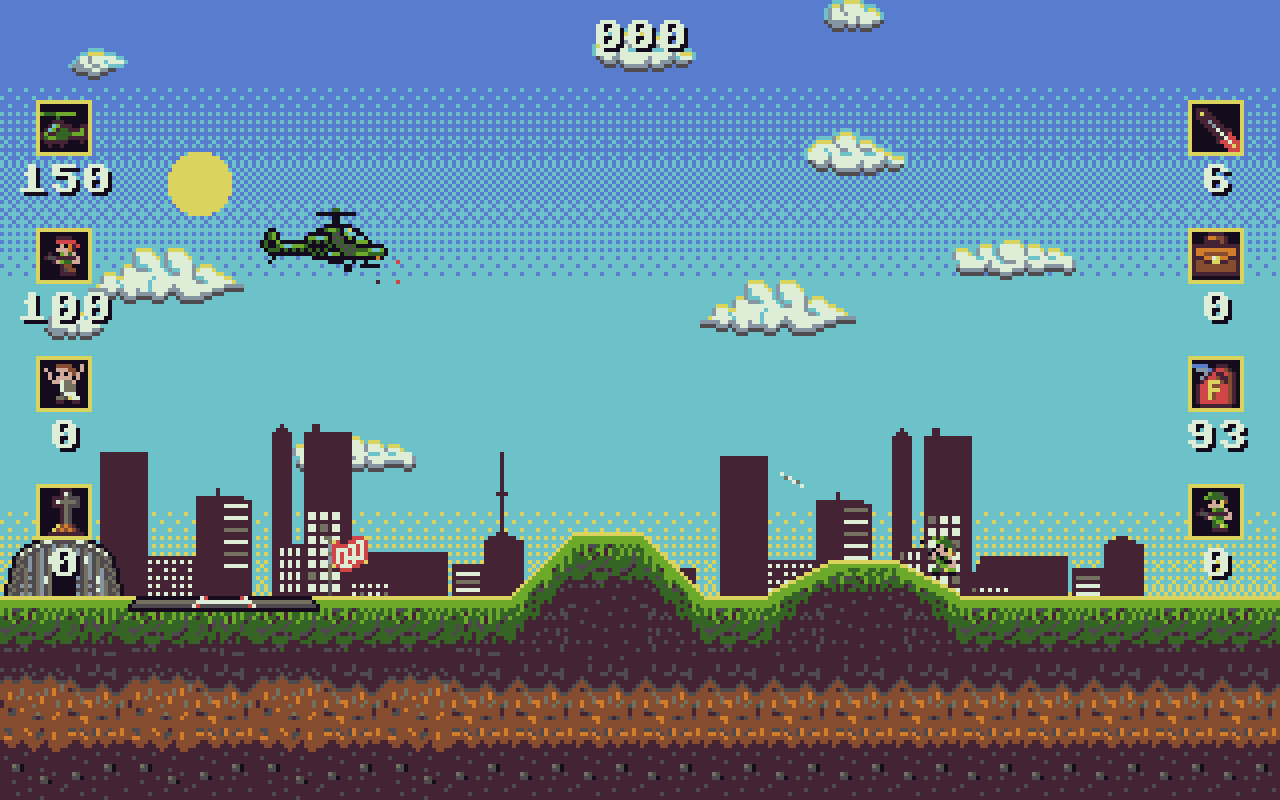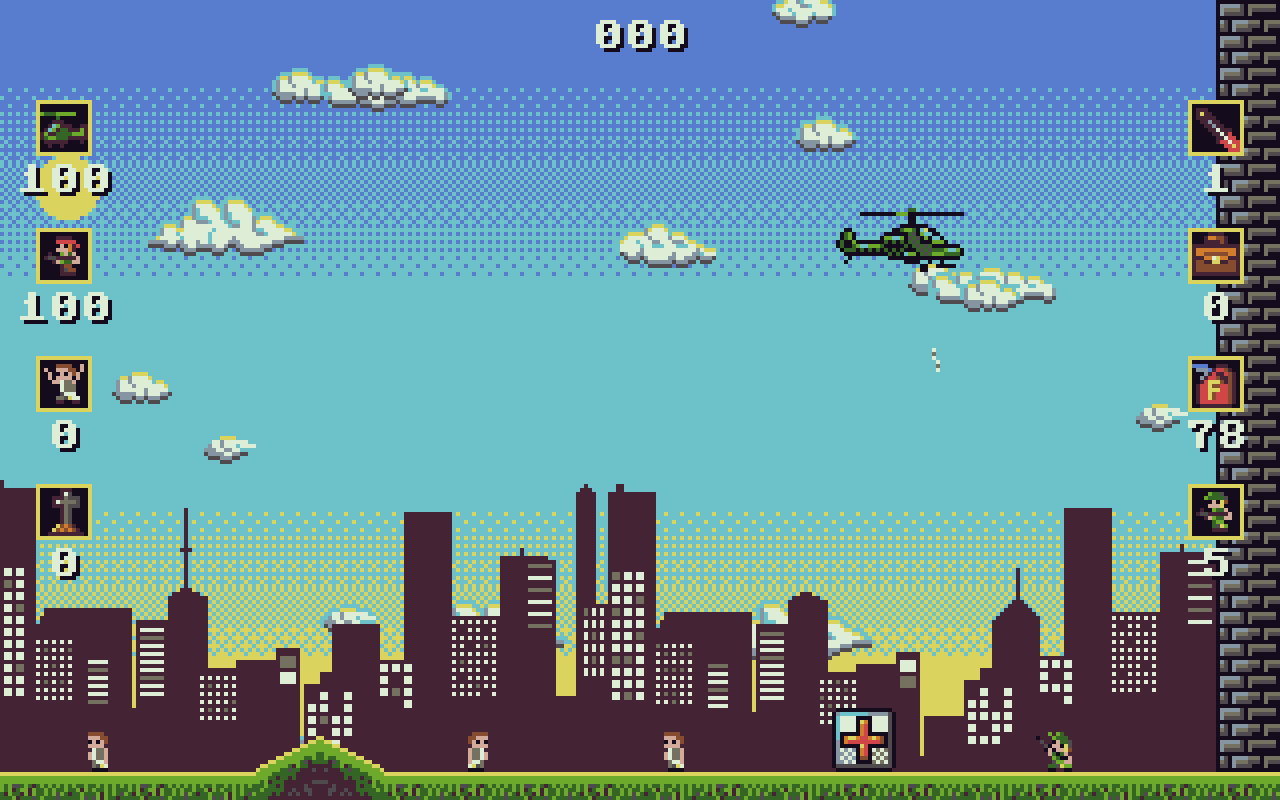-=+=- -=+=- -=+=- -=+=- -=+=- -=+=- -=+=- -=+=- -=+=- -=+=- -=+=- -=+=- -=+=- -=+=- -=+=- -=+=- -=+=- -=+=- -=+=- -=+=- -=+=- -=+=- -=+=- -=+=- -=+=- -=+=- -=+=- -=+=- -=+=- -=+=- (c) WidthPadding Industries 1987 0|79|0 -=+=- -=+=- -=+=- -=+=- -=+=- -=+=- -=+=- -=+=- -=+=- -=+=- -=+=- -=+=- -=+=- -=+=- -=+=- -=+=- -=+=- -=+=- -=+=- -=+=- -=+=- -=+=- -=+=- -=+=- -=+=- -=+=- -=+=- -=+=- -=+=- -=+=-
SoCoder -> Showcase Home -> Shoot 'em Up

therevillsgamesCreated : 20 January 2018

System : Windows
Language : Monkey2

### Get to Da Choppa!

https://therevillsgames.itch.io/get-to-da-choppa
ScreenshotsGet to Da Choppa is my entry for the 'Syntax Bomb' Retro Game competition 2017/18. 320x200 resolution and 16 colours.

Mostly inspired by the Amiga game: Apocalypse.

Developed in Monkey2!

*** TOP SECRET ***

OPERATION: DA CHOPPA

You are a special agent of a top secret organisation. Your mission is to rescue all the hostages and recover the intel.

Good Luck!

Controls (keyboard customisable):

Cursor keys to move

Keyboard Z / Button A = Shoot

Keyboard X / Button B = Seeker Rockets

Keyboard C / Button X = Enter/Exit Choppa

therevillsgames.itch.io/get-to-da-choppa

Sunday, 21 January 2018, 06:56
rockfordLooks greatThursday, 25 January 2018, 04:56
JayenkaiA lovely little gameThursday, 25 January 2018, 17:31
therevillsgamesThanks Jay! And thanks for the little bonus